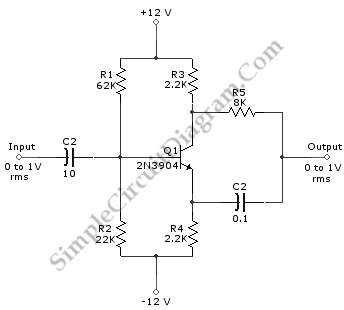# Single Transistor Variable 0-180 Deg Phase Shifter

The schematic diagram below shows a variable 0-180 degree phase shifter circuit. This circuit can provide  phase shifted output (compared with the input) between 0 and 180 degrees for frequencies up to 3MHz with same amplitude (unity gain or gain=1.) This circuit uses inexpensive NPN transistor, 2N3904, but almost any small signal transistor type can be used. Using R5 and C2 values as shown in the schematic diagram, this circuit produces 90° shift for 200 Hz. If you want to change the phase shift amount, we can just vary the values of R5 and C2. Here is the schematic diagram of the circuit: